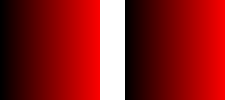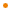# JavaFX: Bringing Rich Experiences To All the Screens Of Your Life

### Overview

The `LinearGradient` class fills a shape with a linear color gradient pattern. The user may specify two or more gradient colors, and this Paint will provide an interpolation between each color.

The application provides an array of `Stop`s specifying how to distribute the colors along the gradient. The `Stop#offset` variable must be the range 0.0 to 1.0 and act like keyframes along the gradient. They mark where the gradient should be exactly a particular color.

If the proportional variable is set to true (the default) then the start and end points of the gradient should be specified relative to the unit square (0.0->1.0) and will be stretched across the shape. If proportional variable is set to false, then the start and end points should be specified as absolute pixel values and the gradient will not be stretched at all.

The two filled rectangles in the example below will render the same. The one on the left uses proportional coordinates (the default) to specify the gradient's end points. The one on the right uses absolute coordinates. Both of them fill the specified rectangle with a horizontal gradient that varies from black to red

the code:

``````import javafx.scene.Group;
import javafx.scene.shape.Rectangle;
import javafx.scene.paint.*;

Group {
content: [
// object bounding box relative (proportional:true, default)
Rectangle {
x: 0 y: 0 width: 100 height: 100
fill: LinearGradient {
startX: 0.0, startY: 0.0, endX: 1.0, endY: 0.0
proportional: true
stops: [ Stop { offset: 0.0 color: Color.BLACK },
Stop { offset: 1.0 color: Color.RED } ]
}
},

// user space relative (proportional:false)
Rectangle {
x: 125 y: 0 width: 100 height: 100
fill: LinearGradient {
startX: 125.0, startY: 0.0, endX: 225.0, endY: 0.0
proportional: false
stops: [ Stop { offset: 0.0 color: Color.BLACK },
Stop { offset: 1.0 color: Color.RED } ]
}
}
]
}``````

produces:Profile: common

### Variable Summary

accessnametypedescription
public-initcycleMethodCycleMethodNO_CYCLE

Defines which of the follwing cycle method is applied to the `LinearGradient`: `CycleMethod.NO_CYCLE`, `CycleMethod.REFLECT`, or `CycleMethod.REPEAT`.

public-initendXNumber1.0

Defines the X coordinate of the gradient axis end point.Defines the X coordinate of the gradient axis end point. If proportional is true (the default), this value specifies a point on a unit square that will be scaled to match the size of the the shape that the gradient fills.

1.0

Profile: common

public-initendYNumber1.0

Defines the Y coordinate of the gradient axis end point.Defines the Y coordinate of the gradient axis end point. If proportional is true (the default), this value specifies a point on a unit square that will be scaled to match the size of the the shape that the gradient fills.

1.0

Profile: common

public-initproportionalBooleantrue

Indicates whether start and end values are proportional or absolute.Indicates whether start and end values are proportional or absolute. If this flag is true, start and end values are defined in a [0..1] space and will be scaled to match the size of the shape that the gradient fills. If this flag is false, then start and end values are absolute coordinates.

true

Profile: common

public-initstartXNumber0.0

Defines the X coordinate of the gradient axis start point.Defines the X coordinate of the gradient axis start point. If proportional is true (the default), this value specifies a point on a unit square that will be scaled to match the size of the the shape that the gradient fills.

0.0

Profile: common

public-initstartYNumber0.0

Defines the Y coordinate of the gradient axis start point.Defines the Y coordinate of the gradient axis start point. If proportional is true (the default), this value specifies a point on a unit square that will be scaled to match the size of the the shape that the gradient fills.

0.0

Profile: common

public-initstopsStop[]empty

A sequence of 2 or more `Stop` values specifying how to distribute the colors along the gradient.A sequence of 2 or more `Stop` values specifying how to distribute the colors along the gradient. These values must be in the range 0.0 to 1.0. They act like keyframes along the gradient: they mark where the gradient should be exactly a particular color.

Each stop in the sequence must have an offset that is greater than the previous stop in the sequence.

empty

Profile: common